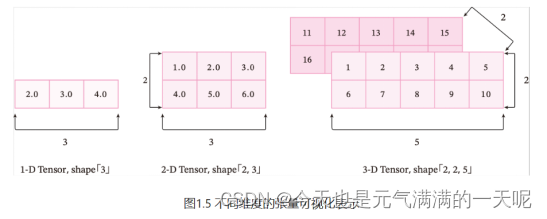# PyTorch学习：关于张量的一些认识

## 一、张量的概念

### 1.张量与NumPy的区分和相似点

#### （1）相似点

Tensor和numpy都能进行基础的数值运算，比如数组间的加减乘除。

Tensor和numpy都可以支持多维数组的操作，比如创建多维数组，还有索引等功能。

#### （2）区别

Tensor：属于pytorch库，主要在深度学习和神经网络中使用；它有自动求导功能，可以方便地进行梯度计算和反向传播；支持CUDA的图形处理器 (GPU) 上运行，并利用GPU的并行计算能力加速深度学习任务。

Numpy：属于python库，主要在数学方面使用；它没有自动求导功能；主要在CPU上运行，不能直接利用GPU加速计算。

### 2.张量与Python中数组列表的区分

Python的列表和元组实际上是在内存中分配的Python对象的集合，并且在内存里，他们不是连续的，而是分散分布的；PyTorch的张量或者NumPy数组通常在内存中存储的是一段连续的、未装箱的C数字类型

### 3.什么是张量## 二、张量的函数

### 1.创建张量

#### （1）指定维度创建张量：分别创建一维张量、二维张量以及N维张量（设N=3）

import torch
Tensor_1=torch.Tensor([1.0,2.0,3.0])
print(Tensor_1)
Tensor_2=torch.Tensor([[1.0,2.0,3.0],[4.0,5.0,6.0]])
print(Tensor_2)
Tensor_3=torch.Tensor([[[1.0,2.0,3.0],[4.0,5.0,6.0],[7.0,8.0,9.0]]])
print(Tensor_3)

#### （2）指定数据值创建张量

import torch
zeros_Tensor=torch.zeros([2,3])#张量数据值都为0
print(zeros_Tensor)
ones_Tensor=torch.ones([2,3])#张量数据值都为1
print(ones_Tensor)
num_Tensor=torch.full([2,3],10)#张量数据值为10
print(num_Tensor)

#### （3）指定区间创建张量

import torch
arange_Tensor = torch.arange(start=0, end=6, step=1)#arange
linspace_Tensor = torch.linspace(start=0, end=6, steps=7)#linspace
print(arange_Tensor)
print(linspace_Tensor)

### 2.张量的属性

#### （1）查看张量属性

#接下来通过创建一个四维中值都为1的张量进行演示：
import torch
Tensor_4=torch.ones(2,3,4,5)
print('张量维度',Tensor_4.ndim)
print('张量每个维度上元素数量',Tensor_4.shape)
print('张量第1维的大小',Tensor_4.shape)
print('张量中全部元素数量', Tensor_4.numel())

#### （2）改变张量的一些属性

# 定义一个shape为[3,2,5]的三维Tensor
import torch
Tensor_3=torch.Tensor([[[1,2,3,4,5],[2,3,4,5,6]],[[3,4,5,6,7],[5,6,7,8,9]],[[6,7,8,9,10],[7,8,9,10,11]]])
print(Tensor_3)
#改变各维度中元素数量
reshape_Tensor=torch.reshape(Tensor_3,[2,5,3])
print(reshape_Tensor)
#改为一维张量
new_Tensor1=Tensor_3.reshape([-1])
new_Tensor2=Tensor_3.reshape([1,5,6])
print(new_Tensor2,new_Tensor2)

ones_Tensor=torch.ones([2,5])
#增加维度
new_Tensor3=torch.unsqueeze(ones_Tensor,dim=0)
new_Tensor4=torch.unsqueeze(ones_Tensor,dim=1)
print(new_Tensor3.shape,new_Tensor4.shape)

#### （3）查看、改变张量的数据类型

import torch
# 查看张量的数据类型
num_Tensor=torch.full([2,3],10)#张量数据值为10
print('num_Tensor',num_Tensor)
print('num_Tensor的张量类型',num_Tensor.dtype)
Tensor_1=torch.Tensor([0,1,2,3,4,5,6,7,8,9])
print('Tensor_1',Tensor_1)
print('Tensor_1的张量类型',Tensor_1.dtype)
#改变张量的数据类型
int64_Tensor = Tensor_1.type(torch.int64)
print('改变后Tensor_1的数据类型:', int64_Tensor.dtype)

### 3.张量与Numpy数组转换

import torch
import numpy as np
num_Tensor=torch.full([2,3],10)#张量数据值为10
print('num_Tensor',num_Tensor)
print('num_Numpy',num_Tensor.numpy())

### 4.张量的访问

#### （1）访问张量，进行索引、切片

import torch
#访问1维张量
Tensor_1=torch.Tensor([0,1,2,3,4,5,6,7,8,9])
print('Tensor_1',Tensor_1)
print('访问第一个元素',Tensor_1.item())
print('访问最后一个元素',Tensor_1[-1].item())
print('访问所有元素',Tensor_1[:])
print('访问前三个',Tensor_1[:3])
print('每隔三个元素访问一次',Tensor_1[::3])
numpy_Tensor=Tensor_1.numpy()
print('倒着访问',numpy_Tensor[::-1])
#访问二维张量
Tensor_2=torch.Tensor([[1,2,3,4,5],[6,7,8,9,0]])
print('Tensor_2',Tensor_2)
print('第一行',Tensor_2)
print('第一行',Tensor_2[0,:])
print('第一列',Tensor_2[:,0])
print('最后一列',Tensor_2[:,-1])
print('整体',Tensor_2[:])
print('第一行第二列',Tensor_2[0,1])

#### （2）修改张量中数据值

import torch
ones_Tensor_2 = torch.ones([2, 3], dtype=torch.float32)
print(ones_Tensor_2)
ones_Tensor_2 = 0
print('修改第一维都为0:', ones_Tensor_2)
ones_Tensor_2 = 2.1
print('修改第一维都为2.1:', ones_Tensor_2)
ones_Tensor_2[...] = 3
print('修改整个张量值为3:', ones_Tensor_2)

x[]就可以进行各种方式的访问，比如x[:]全访问、x[:3]访问前三个、x[-1]访问最后一个。但是需要注意的是，想要逆序访问，需要先将tensor转为numpy，然后在进行[::-1]，才能进行逆序访问。

### 5.张量的运算

#### （1）数学运算

import torch
#数学运算
x = torch.tensor([1.1, 2.2], dtype=torch.float32)
y = torch.tensor([3.3, 4.4], dtype=torch.float32)
print('四舍五入',x.round())
print('取倒数',x.reciprocal())
print('幂运算',x.pow(y))
print('最大值',x.max())
print('正弦',x.sin())
print('求和',x.sum())
print('计算平方',x.sqrt())

#### （2）逻辑运算

#逻辑运算
print('x',x)
print('y',y)
print('判断两个张量的每个元素是否相等',x.eq(y))
print('判断两个张量的全部元素是否相等',x.equal(y))
print('判断每个元素是否小于y',x.lt(y))
print('判断两个张量是否接近',x.allclose(y))

#### （3）矩阵运算

#矩阵运算
x = torch.tensor([[1, 2, 3],[4, 5, 6]])
y=torch.tensor([[3,4,5],[6,7,8]])
result1 = torch.transpose(x, -2, -1)
print('交换第一维和第二维的数据',result1)
result2=torch.mul(x,y)
print('矩阵乘法',result2)
print('转置',x.t())

10-281147
08-19229
08-0766
02-211995
03-13420
10-03717
07-251655
01-271720
05-151011
02-06169
06-121148
12-05725
08-11676
02-281152
07-11540
11-07808
10-271513
09-28238

### “相关推荐”对你有帮助么？

•非常没帮助
•没帮助
•一般
•有帮助
•非常有帮助被折叠的  条评论 为什么被折叠?到【灌水乐园】发言¥1 ¥2 ¥4 ¥6 ¥10 ¥20获取中扫码支付点击重新获取扫码支付1.余额是钱包充值的虚拟货币，按照1:1的比例进行支付金额的抵扣。
2.余额无法直接购买下载，可以购买VIP、付费专栏及课程。余额充值